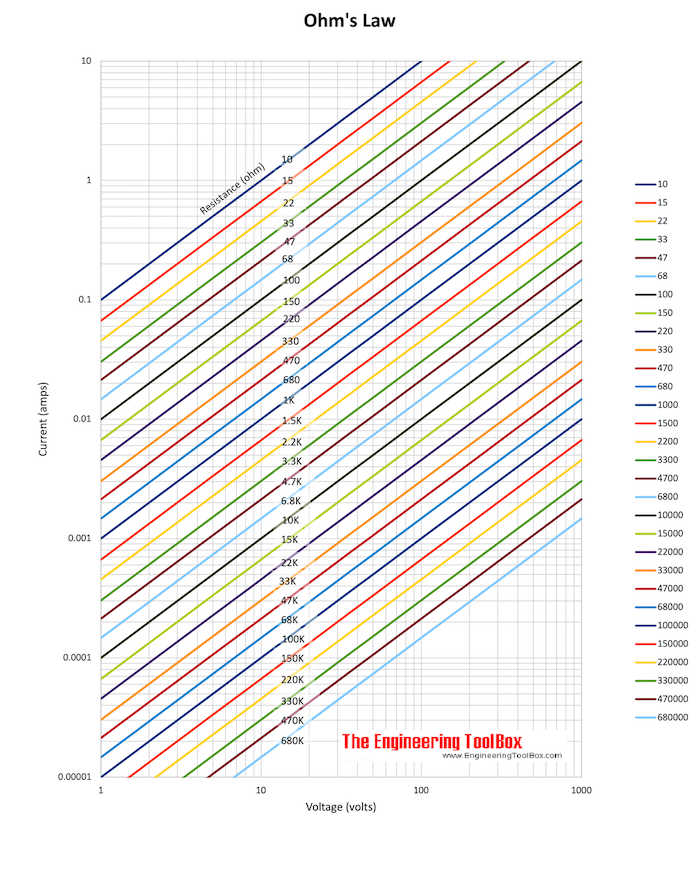Engineering ToolBox - Resources, Tools and Basic Information for Engineering and Design of Technical Applications!

# Ohm's Law

## The relation between voltage, current and electrical resistance.

Ohm's law states that

"the current through a conductor between two points is directly proportional to the potential difference or voltage across the two points, and inversely proportional to the resistance between them".

Ohm's law can be expressed as

I = U / R                     (1)

where

I = current (ampere, A)

U = electrical potential (volts, V)

R = resistance (ohms, Ω)

#### Example - Ohm's law

A 12 volt battery supplies power to a resistance of 18 ohms. The current in the elctrical circuit can be calculated as

I = (12 volts) / (18 ohm)

= 0.67 ampere### Equivalent Expressions of Ohm's Law

Ohm's law (1) can also be expressed as

U = R I                         (2)

or

R = U / I                       (3)#### Example - Electric Circuit Resistance

A current of 1 ampere is flowing through a 230 V electric circuit. From the diagram above this indicates resistance

R ≈ 220 Ω

This can alternatively be calculated with Ohm's law

R = (230 V) / (1 A)

= 230 Ω

#### Example - Ohm's Law and Multiples and Submultiples

Currents, voltages and resistances in electric circuits may often be very small or very large - so multiples and submultiples are often used.

The voltage required applied to a 3.3 kΩ resistor to generate a current of 20 mA can be calculated as

U = (3.3 kΩ) (1000 Ω/kΩ) (20 mA) (10-3 A/mA)

= 66 V

#### Electric Resistance Nomogram

The default values in the nomogram above indicates 230 volts, resistance 24 ohm and current 10 amps.

### Power

Electric power can be expressed as

P = U I

= R I2

= U2 / R                     (4)

where

P = electrical power (watts, W)

#### Example - Power Consumed

The power consumed in the 12V electrical circuit above can be calculated as

P = (12 volts)2 / (18 ohm)

=  8 W

#### Example - Power and Electrical Resistance

A 100 W electric light bulb is connected to a 230 V supply. The current flowing can be calculated by reorganizing (4) to

I = P / U

= (100 W) / (230 V)

= 0.43 ampere

The resistance can be calculated by reorganizing (4) to

R = U2 / P

= (230 V)2 / (100 W)

= 529 Ω

#### Electric Power Nomogram

This nomogram can be used to estimate power vs. voltage and ampere.

The default values in the nomogram above indicates 240 volts, resistance 10 amps and power 2.4 kW for DC or single phase AC - and 4 kW for three phase AC.

## Related Topics

• ### Electrical

Electrical units, amps and electrical wiring, wire gauge and AWG, electrical formulas and motors.

## Related Documents

• ### Aluminum Conductor Characteristics

Characteristics of all-aluminum conductors (AAC).
• ### Copper Wire - Electrical Resistance vs. Gauge

Gauge, weight, circular mils and electrical resistance in copper wire.
• ### Electric Circuit Diagram - Drawing Template

Online shareable electric circuit diagram.
• ### Electric Circuits Voltage Drop

Ohm's law and voltage drop in electric circuits.
• ### Electrical Formulas

Commonly used electrical formulas like Ohms Law and more.
• ### Electrical Parallel Circuits

Resistance, voltage and current in electrical parallel circuits.
• ### Electrical Series Circuits

Voltage and current in electrical series circuits.
• ### Electrical Units

Definition of common electrical units - like Ampere, Volt, Ohm, Siemens.
• ### EV - Electric Vehicles Range vs. State of Charge (SOC) Calculator

Electric vehicle range vs. battery size, SOC - state of charge and energy consumption.
• ### Nickel-Chromium Heater Wire - Temperature Rise vs. Amps

Electric resistance vs. temperature rise for Nickel-Chromium electrical heater wire.
• ### Resistance vs. Conductance

The reciprocal of electrical resistance is conductance.
• ### Resistivity and Conductivity - Temperature Coefficients Common Materials

Resistivity, conductivity and temperature coefficients for common materials like silver, gold, platinum, iron and more..
• ### Resistors - Color Codes Calculator

Color codes for fixed resistors - values and tolerances - online calculator.
• ### Resistors - Standard Values

Preferred number series for resistors.

## Engineering ToolBox - SketchUp Extension - Online 3D modeling!

Add standard and customized parametric components - like flange beams, lumbers, piping, stairs and more - to your Sketchup model with the Engineering ToolBox - SketchUp Extension - enabled for use with older versions of the amazing SketchUp Make and the newer "up to date" SketchUp Pro . Add the Engineering ToolBox extension to your SketchUp Make/Pro from the Extension Warehouse !

We don't collect information from our users. More about

## Citation

• The Engineering ToolBox (2010). Ohm's Law. [online] Available at: https://www.engineeringtoolbox.com/ohms-law-d_1658.html [Accessed Day Month Year].

Modify the access date according your visit.

9.29.12